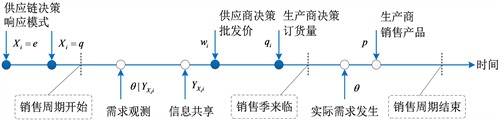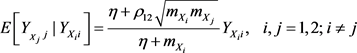﻿ 水平竞争条件下的供应链模式研究

水平竞争条件下的供应链模式研究The Response Mode of Supply Chain under Horizontal Competition

Abstract: There are two types of response modes from which the supply chains can choose: responsive supply chain and efficient supply chain. This paper builds a model of single supply chain and of two supply chains under horizontal competition. Then the demand information accuracy is mathematically described and the interaction between information accuracy and production cost is quantified. On this basis, this paper studies the influence of cost effect and information effect on the mode selection of the supply chain. We show that when there is only one supply chain and ignoring the cost factor, responsive supply chain is the unique equilibrium. When there are two competing supply chains, the coupling effect between their demand information should be taken into consideration besides the cost effect and precision effect. The results suggest that because of the coupling of information, responsive supply chain isn’t always the equilibrium strategy even if the production cost is not high or the information is more accurate. This is because the competition intensity will be intensified when competitors adopt the same supply chain mode, which will affect the overall profits of the supply chain on the contrary.

1. 引言

21世纪的企业必须应对各种行业竞争及市场变化，以满足顾客对高质量、低价格产品的需求。为此目的，企业需要对顾客独特并快速变化的需求做出响应，其中一项供应链管理措施即对企业所处的供应链进行模式的决策。目前的供应链模式主要有响应型供应链和效率型供应链等。企业在选择供应链模式时往往面临两个方面的决策，一是采用较长的生产准备期，从而降低生产成本，但是准备提前期越长，对市场需求的把握就越不准确，容易产生供需不匹配；另一方面若缩短生产准备期，则可以在需求明朗时再精准地响应市场，但是由于生产期短，往往会面临比较高的生产成本。因此企业在决策采取响应型或效率型供应链时，会面临降低生产成本与采集精准需求信息的权衡。

2. 相关研究

3. 问题描述

$b{q}_{i}+\frac{{c}_{{X}_{i}}}{2}{q}_{i}^{2}$

3.1. 博弈时序

1) 两条供应链首先选择各自模式 $\left({X}_{i},{X}_{j}\right)$，同时确定其相应的生产成本。

2) 销售季来临前，双方根据各自的供应链模式，通过观测得到需求信息 ${Y}_{{X}_{i}i},{Y}_{{X}_{j}j}$，并在己方供应链Figure 1. Game sequence diagram

3) 各供应商制定配件的批发价 ${w}_{i},{w}_{j}$，并向己方生产商发布。

4) 各生产商决策订货量 ${q}_{i},{q}_{j}$，供应商按照生产商的订货量满足相应配件需求。

5) 销售季来临，实际需求发生，各生产商按市场统一价格p出售所有产品；销售周期结束，各供应

3.2. 需求信息结构

$E\left[\theta |{Y}_{{X}_{i}i}\right]=\frac{\eta }{\eta +{m}_{{X}_{i}}}{Y}_{{X}_{i}i}$ (1)

$E\left[{Y}_{{}_{{X}_{j}}j}|{Y}_{{X}_{i}i}\right]=\frac{\eta +\rho {m}_{{X}_{i}}}{\eta +{m}_{{X}_{i}}}{Y}_{{X}_{i}i},\text{\hspace{0.17em}}\text{\hspace{0.17em}}\text{\hspace{0.17em}}i,j=1,2;\text{\hspace{0.17em}}i\ne j$ (2)(3)

4. 单条供应链的模式决策

${q}^{\text{*}}\left(w\right)=\frac{a+E\left[\theta |{Y}_{X}\right]-w}{2}$ (4)

${w}^{\text{*}}=\frac{2+{c}_{X}}{4+{c}_{X}}\left(a+E\left[\theta |{Y}_{X}\right]\right)$ (5)

${q}^{*}\left({Y}_{X}\right)=\frac{a}{4+{c}_{X}}+\frac{\eta }{\left(4+{c}_{X}\right)\left(\eta +{m}_{X}\right)}{Y}_{X}$

${\Pi }_{M}={\stackrel{¯}{\Pi }}_{M}+\frac{{\eta }^{2}}{{\left(4+{c}_{X}\right)}^{2}\left(\eta +{m}_{X}\right)}$${\Pi }_{S}={\stackrel{¯}{\Pi }}_{S}+\frac{{\eta }^{2}}{2\left(4+{c}_{X}\right)\left(\eta +{m}_{X}\right)}$$\Pi =\stackrel{¯}{\Pi }+\frac{\left(6+{c}_{X}\right){\eta }^{2}}{2{\left(4+{c}_{X}\right)}^{2}\left(\eta +{m}_{X}\right)}$

a) 当 $T\left(q\right)>T\left(e\right)$ 时， ${\Pi }^{q}>{\Pi }^{e}$，此时 $X=q$ 即响应型供应链为最优供应链模式；

b) 当 $T\left(q\right) 时， ${\Pi }^{q}<{\Pi }^{e}$，此时 $X=e$ 即效率型供应链为最优供应链模式。

a) $\partial T\left(X\right)/\partial {m}_{X}<0$ 恒成立，即当信息精度增加( ${m}_{X}$ 减小)时， $T\left(X\right)$ 取值增大，对期望收益起到正向作用；

b) $\partial T\left(X\right)/\partial {c}_{X}<0$ 恒成立，即当生产成本 ${c}_{X}$ 增大时， $T\left(X\right)$ 取值减小，对期望收益起负向作用。

5. 两条供应链的水平竞争模型

$p=a+\theta -{q}_{i}-{q}_{j},\text{\hspace{0.17em}}\text{\hspace{0.17em}}i\ne j$

${\Pi }_{{M}_{i}}=\left(a+E\left[\theta |{Y}_{{X}_{i}i}\right]-{q}_{i}-E\left[{q}_{j}|{Y}_{{X}_{i}i}\right]-{w}_{i}\right){q}_{i}$

${q}_{i}^{*}\left({q}_{j},{w}_{i}\right)=\frac{a+E\left[\theta |{Y}_{{X}_{i}i}\right]-E\left[{q}_{j}|{Y}_{{X}_{i}i}\right]-{w}_{i}}{2}$ (6)

${\Pi }_{{S}_{i}}={w}_{i}{q}_{i}\left({q}_{j},{w}_{i}\right)-\frac{{c}_{{X}_{i}}}{2}{\left({q}_{i}\left({q}_{j},{w}_{i}\right)\right)}^{2}$

 (7)

${q}_{i}^{*}\left({q}_{j},{w}_{i}^{*}\left({q}_{j}\right)\right)=\frac{1}{4+{c}_{{X}_{i}}}\left(a+E\left[\theta |{Y}_{{X}_{i}i}\right]-E\left[{q}_{j}|{Y}_{{X}_{i}i}\right]\right),\text{\hspace{0.17em}}\text{\hspace{0.17em}}i\ne j$ (8)

${\Pi }_{{M}_{i}}^{*}={\left({q}_{i}^{*}\right)}^{2}$ (9)

${\Pi }_{{S}_{i}}^{*}=\frac{4+{c}_{{X}_{i}i}}{2}{\left({q}_{i}^{*}\right)}^{2}$ (10)

5.1. 生产商订货量决策

${q}_{i}^{*}\left({Y}_{{X}_{i}i}\right)={\stackrel{¯}{q}}_{i}+{C}_{i}^{{X}_{i},{X}_{j}}{Y}_{{X}_{i}i}$

${C}_{i}^{e,e}=\frac{\eta }{{h}_{e}\left(\eta +{m}_{e}\right)+\eta +\rho {m}_{e}}$${C}_{i}^{q,q}=\frac{\eta }{{h}_{q}\left(\eta +{m}_{q}\right)+\eta +\rho {m}_{q}}$

${C}_{i}^{e,q}=\frac{\eta \left[{h}_{q}\left(\eta +{m}_{q}\right)-\left(\eta +{\rho }_{12}\sqrt{{m}_{e}{m}_{q}}\right)\right]}{{h}_{e}{h}_{q}\left(\eta +{m}_{e}\right)\left(\eta +{m}_{q}\right)-{\left(\eta +{\rho }_{12}\sqrt{{m}_{e}{m}_{q}}\right)}^{2}}$${C}_{i}^{q,e}=\frac{\eta \left[{h}_{e}\left(\eta +{m}_{e}\right)-\left(\eta +{\rho }_{12}\sqrt{{m}_{e}{m}_{q}}\right)\right]}{{h}_{e}{h}_{q}\left(\eta +{m}_{e}\right)\left(\eta +{m}_{q}\right)-{\left(\eta +{\rho }_{12}\sqrt{{m}_{e}{m}_{q}}\right)}^{2}}$

5.2. 供应商批发价决策

${w}_{i}^{*}\left({Y}_{{X}_{i}i}\right)=\left({h}_{{X}_{i}}-2\right)\left(\frac{{h}_{{X}_{j}}-1}{{h}_{{X}_{i}}{h}_{{X}_{j}}-1}a+{C}_{i}^{{X}_{i},{X}_{j}}{Y}_{{X}_{i}i}\right)$

5.3. 供应链期望收益

1描述了两条供应链在各自选择的模式 $\left({X}_{i},{X}_{j}\right)$ 下，供应链各成员及整体的期望收益。

${\Pi }_{{M}_{i}}^{{X}_{i},{X}_{j}}={\stackrel{¯}{\Pi }}_{{M}_{i}}+\left(\eta +{m}_{{X}_{i}}\right){\left({C}_{i}^{{X}_{i},{X}_{j}}\right)}^{2}$${\Pi }_{{S}_{i}}^{{X}_{i},{X}_{j}}={\stackrel{¯}{\Pi }}_{{S}_{i}}+\frac{{h}_{{X}_{i}}\left(\eta +{m}_{{X}_{i}}\right){\left({C}_{i}^{{X}_{i},{X}_{j}}\right)}^{2}}{2}$

${\Pi }_{i}^{{X}_{i},{X}_{j}}={\stackrel{¯}{\Pi }}_{i}+\frac{\left(2+{h}_{{X}_{i}}\right)\left(\eta +{m}_{{X}_{i}}\right){\left({C}_{i}^{{X}_{i},{X}_{j}}\right)}^{2}}{2}$

${\Pi }_{{M}_{i}}^{{X}_{i},{X}_{j}}={\Pi }_{{M}_{i}}^{{X}_{i}}\left({C}_{i}^{{X}_{i},{X}_{j}}\right)$${\Pi }_{{S}_{i}}^{{X}_{i},{X}_{j}}={\Pi }_{{S}_{i}}^{{X}_{i}}\left({C}_{i}^{{X}_{i},{X}_{j}}\right)$${\Pi }_{i}^{{X}_{i},{X}_{j}}={\Pi }_{i}^{{X}_{i}}\left({C}_{i}^{{X}_{i},{X}_{j}}\right)$

5.4. 供应链的最优模式决策

${V}_{i}^{{X}_{j}}={\Pi }_{i}^{q,{X}_{j}}-{\Pi }_{i}^{e,{X}_{j}}={\Pi }_{i}^{q}\left({C}_{i}^{q,{X}_{j}}\right)-{\Pi }_{i}^{e}\left({C}_{i}^{e,{X}_{j}}\right)$

${V}_{i}^{{X}_{j}}=\left[{\Pi }_{i}^{q}\left({C}_{i}^{e,{X}_{j}}\right)-{\Pi }_{i}^{e}\left({C}_{i}^{e,{X}_{j}}\right)\right]+\left[{\Pi }_{i}^{q}\left({C}_{i}^{q,{X}_{j}}\right)-{\Pi }_{i}^{q}\left({C}_{i}^{e,{X}_{j}}\right)\right]$ (11)

a) 第一项始终为负，对 ${V}_{i}^{{X}_{j}}$ 增值起负向作用，即仅考虑部分信息精度而忽略信息耦合度作用，将对供应链i造成损失，阻碍其通过响应型供应链模式增加收益；

b) 当 ${X}_{j}=q$ 时，若相关系数间满足 ${\rho }_{12}<\sqrt{{m}_{q}/{m}_{e}}\rho$，存在临界值 ${\delta }^{\text{*}}\left(0<{\delta }^{*}<1\right)$，当 ${m}_{q}<{\delta }^{*}{m}_{e}$ 时，第二项为正，当 ${m}_{q}>{\delta }^{\text{*}}{m}_{e}$ 时，第二项为负，当 ${m}_{q}={\delta }^{*}{m}_{e}$ 时，第二项的综合作用为0；当 ${X}_{j}=e$ 时，第

a) 当需求信息的相关系数间满足 ${\rho }_{12}<\sqrt{{m}_{q}/{m}_{e}}\rho$ 时，存在临界值 ${\xi }^{*}\left(0<{\xi }^{*}<1\right)$，当 ${m}_{q}<{\xi }^{*}{m}_{e}$ 时， ${V}_{i}^{q}>0$，此时 $\left({X}_{i},{X}_{j}\right)=\left(q,q\right)$ 为最优供应链模式；当 ${m}_{q}>{\xi }^{*}{m}_{e}$ 时， ${V}_{i}^{q}<0$，此时 $\left({X}_{i},{X}_{j}\right)=\left(e,q\right)$ 为最优供应链模式；当 ${m}_{q}={\xi }^{\text{*}}{m}_{e}$ 时， $\left({X}_{i},{X}_{j}\right)=\left(q,q\right)$$\left({X}_{i},{X}_{j}\right)=\left(e,q\right)$ 都为均衡供应链模式。

b) ${V}_{i}^{e}>0$ 恒成立，此时 $\left({X}_{i},{X}_{j}\right)=\left(q,e\right)$ 为最优供应链模式。

6. 结论

 Gunasekaran, A., Lai, K.H. and Cheng, T.C.E. (2008) Responsive Supply Chain: A Competitive Strategy in a Networked Economy. Omega, 36, 549-564.
https://doi.org/10.1016/j.omega.2006.12.002

 Randall, T.R., Morgan, R.M. and Morton, A.R. (2003) Efficient versus Responsive Supply Chain Choice: An Empirical Examination of Influential Factors. Journal of Product Innovation Management, 20, 430-443.
https://doi.org/10.1111/1540-5885.00041

 Fu, K., Xu, J. and Miao, Z. (2013) Newsvendor with Multiple Options of Expe-diting. European Journal of Operational Research, 226, 94-99.
https://doi.org/10.1016/j.ejor.2012.10.039

 杨文胜, 马士华, 李莉. 基于供应链响应时间的协同计划模型[J]. 预测, 2004, 23(5): 52-56.

 张英, 王乐乐. 基于平衡计分卡的响应型供应链绩效评价指标体系设计[J]. 物流技术, 2013, 32(17): 374-376.

 Zhang, H. (2002) Vertical Information Exchange in a Supply Chain with Duopoly Retailers. Production and Operations Management, 11, 531-546.
https://doi.org/10.1111/j.1937-5956.2002.tb00476.x

 Wu, X. and Zhang, F. (2014) Home or Overseas? An Analysis of Sourcing Strategies under Competition. Management Science, 60, 1223-1240.
https://doi.org/10.1287/mnsc.2013.1823

 Ha, A.Y., Tong, S. and Zhang, H. (2011) Sharing Demand Information in Competing Supply Chains with Production Diseconomies. Management Science, 57, 566-581.
https://doi.org/10.1287/mnsc.1100.1295

 宋华明, 杨慧, 罗建强. 基于Bayes需求预测更新的供应链合作策略研究[J]. 管理工程学报, 2011, 25(2): 220-227.

 Anand, K.S. and Goyal, M. (2009) Strategic Infor-mation Management under Leakage in a Supply Chain. Management Science, 55, 438-452.
https://doi.org/10.1287/mnsc.1080.0930

Top